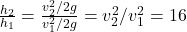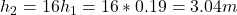## When a garden hose with an output diameter of 20 mmmm is directed straight upward, the stream of water rises to a height of 0.19 mm . You th

Question

When a garden hose with an output diameter of 20 mmmm is directed straight upward, the stream of water rises to a height of 0.19 mm . You then use your thumb to partially cover the output opening so that its diameter is reduced to 10 mmmm. Part A How high does the water rise now? Ignore drag and assume that the smaller opening you create with your thumb is circular.

in progress 0
2 months 2021-08-30T15:33:51+00:00 1 Answers 0 views 0

3.04 m

Explanation:

20 mm = 0.02 m

10 mm = 0.01 m

When the water escape from the hose opening with a velocity v to rise up to a height of 0.19m, its potential energy is converted from kinetic energy: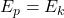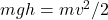where m is the mass and h is the vertical distance traveled, and g = 9.81m/s2 is the gravitational acceleration

We can divide both sides by m and get: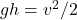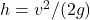(1)

When you cover part of the hose and reduce the cross sectional area, water velocity increases: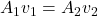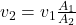As the area of the circular opening is: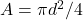where d is the diameter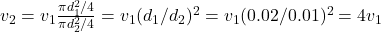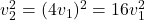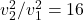From equation (1) we have the following ratio